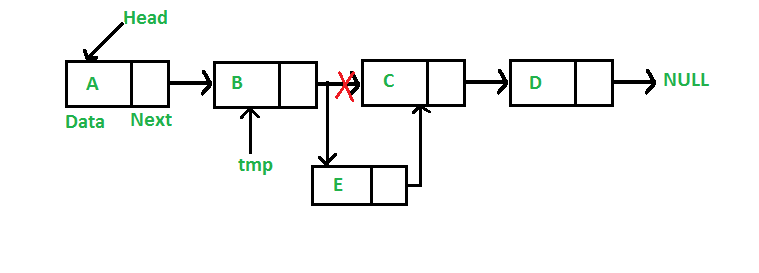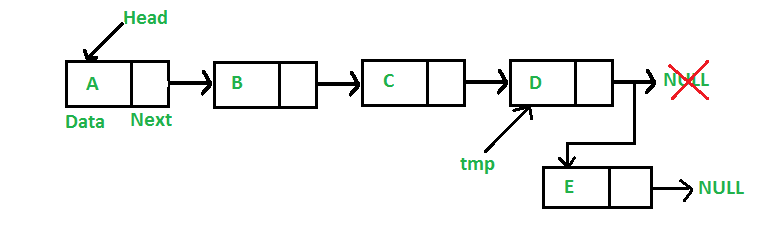Open In App
Related Articles

Given a Linked List, the task is to insert a new node in this given Linked List at the following positions:

• At the front of the linked list
• After a given node.
• At the end of the linked list.

## How to Insert a Node at the Front/Beginning of Linked List

Approach:

To insert a node at the start/beginning/front of a Linked List, we need to:

Below is the implementation of the approach:

## C++

 `// Given a reference (pointer to pointer)``// to the head of a list and an int,``// inserts a new node on the front of``// the list.``void` `push(Node** head_ref, ``int` `new_data)``{` `    ``// 1. allocate node``    ``Node* new_node = ``new` `Node();` `    ``// 2. put in the data``    ``new_node->data = new_data;` `    ``// 3. Make next of new node as head``    ``new_node->next = (*head_ref);` `    ``// 4. Move the head to point to``    ``// the new node``    ``(*head_ref) = new_node;``}`

## C

 `/* Given a reference (pointer to pointer) to the head of a``   ``list``   ``and an int,  inserts a new node on the front of the list.`` ``*/``void` `push(``struct` `Node** head_ref, ``int` `new_data)``{``    ``/* 1. allocate node */``    ``struct` `Node* new_node``        ``= (``struct` `Node*)``malloc``(``sizeof``(``struct` `Node));` `    ``/* 2. put in the data  */``    ``new_node->data = new_data;` `    ``/* 3. Make next of new node as head */``    ``new_node->next = (*head_ref);` `    ``/* 4. move the head to point to the new node */``    ``(*head_ref) = new_node;``}`

## Java

 `/* This function is in LinkedList class. Inserts a``   ``new Node at front of the list. This method is``   ``defined inside LinkedList class shown above */``public` `void` `push(``int` `new_data)``{``    ``/* 1 & 2: Allocate the Node &``              ``Put in the data*/``    ``Node new_node = ``new` `Node(new_data);` `    ``/* 3. Make next of new Node as head */``    ``new_node.next = head;` `    ``/* 4. Move the head to point to new Node */``    ``head = new_node;``}`

## Python3

 `# This function is in LinkedList class``# Function to insert a new node at the beginning``def` `push(``self``, new_data):` `    ``# 1 & 2: Allocate the Node &``    ``# Put in the data``    ``new_node ``=` `Node(new_data)` `    ``# 3. Make next of new Node as head``    ``new_node.``next` `=` `self``.head` `    ``# 4. Move the head to point to new Node``    ``self``.head ``=` `new_node`

## C#

 `/* Inserts a new Node at front of the list. */``public` `void` `push(``int` `new_data)``{``    ``/* 1 & 2: Allocate the Node &``               ``Put in the data*/``    ``Node new_node = ``new` `Node(new_data);` `    ``/* 3. Make next of new Node as head */``    ``new_node.next = head;` `    ``/* 4. Move the head to point to new Node */``    ``head = new_node;``}`

## Javascript

 ``

Complexity Analysis:

• Time Complexity: O(1), We have a pointer to the head and we can directly attach a node and change the pointer. So the Time complexity of inserting a node at the head position is O(1) as it does a constant amount of work.
• Auxiliary Space: O(1)

Refer this post for detailed implementation of the above approach.

## How to Insert a Node after a Given Node in Linked List

Approach:

To insert a node after a given node in a Linked List, we need to:

• Check if the given node exists or not.
• If it do not exists,
• terminate the process.
• If the given node exists,
• Make the element to be inserted as a new node
• Change the next pointer of given node to the new node
• Now shift the original next pointer of given node to the next pointer of new nodeBelow is the implementation of the approach:

## C++

 `// Given a node prev_node, insert a``// new node after the given``// prev_node``void` `insertAfter(Node* prev_node, ``int` `new_data)``{``    ``// 1. Check if the given prev_node is NULL``    ``if` `(prev_node == NULL) {``        ``cout << ``"The given previous node cannot be NULL"``;``        ``return``;``    ``}` `    ``// 2. Allocate new node``    ``Node* new_node = ``new` `Node();` `    ``// 3. Put in the data``    ``new_node->data = new_data;` `    ``// 4. Make next of new node as``    ``// next of prev_node``    ``new_node->next = prev_node->next;` `    ``// 5. move the next of prev_node``    ``// as new_node``    ``prev_node->next = new_node;``}`

## C

 `/* Given a node prev_node, insert a new node after the given``prev_node */``void` `insertAfter(``struct` `Node* prev_node, ``int` `new_data)``{``    ``/*1. check if the given prev_node is NULL */``    ``if` `(prev_node == NULL) {``        ``printf``(``"the given previous node cannot be NULL"``);``        ``return``;``    ``}` `    ``/* 2. allocate new node */``    ``struct` `Node* new_node``        ``= (``struct` `Node*)``malloc``(``sizeof``(``struct` `Node));` `    ``/* 3. put in the data */``    ``new_node->data = new_data;` `    ``/* 4. Make next of new node as next of prev_node */``    ``new_node->next = prev_node->next;` `    ``/* 5. move the next of prev_node as new_node */``    ``prev_node->next = new_node;``}`

## Java

 `/* This function is in LinkedList class.``Inserts a new node after the given prev_node. This method is``defined inside LinkedList class shown above */``public` `void` `insertAfter(Node prev_node, ``int` `new_data)``{``    ``/* 1. Check if the given Node is null */``    ``if` `(prev_node == ``null``) {``        ``System.out.println(``            ``"The given previous node cannot be null"``);``        ``return``;``    ``}` `    ``/* 2. Allocate the Node &``    ``3. Put in the data*/``    ``Node new_node = ``new` `Node(new_data);` `    ``/* 4. Make next of new Node as next of prev_node */``    ``new_node.next = prev_node.next;` `    ``/* 5. make next of prev_node as new_node */``    ``prev_node.next = new_node;``}`

## Python3

 `# This function is in LinkedList class.``# Inserts a new node after the given prev_node. This method is``# defined inside LinkedList class shown above */``def` `insertAfter(``self``, prev_node, new_data):` `    ``# 1. check if the given prev_node exists``    ``if` `prev_node ``is` `None``:``        ``print``(``"The given previous node must inLinkedList."``)``        ``return` `    ``# 2. Create new node &``    ``# 3. Put in the data``    ``new_node ``=` `Node(new_data)` `    ``# 4. Make next of new Node as next of prev_node``    ``new_node.``next` `=` `prev_node.``next` `    ``# 5. make next of prev_node as new_node``    ``prev_node.``next` `=` `new_node`

## C#

 `/* Inserts a new node after the given prev_node. */``public` `void` `insertAfter(Node prev_node, ``int` `new_data)``{``    ``/* 1. Check if the given Node is null */``    ``if` `(prev_node == ``null``) {``        ``Console.WriteLine(``"The given previous node"``                          ``+ ``" cannot be null"``);``        ``return``;``    ``}` `    ``/* 2 & 3: Allocate the Node &``            ``Put in the data*/``    ``Node new_node = ``new` `Node(new_data);` `    ``/* 4. Make next of new Node as``                ``next of prev_node */``    ``new_node.next = prev_node.next;` `    ``/* 5. make next of prev_node``                    ``as new_node */``    ``prev_node.next = new_node;``}`

## Javascript

 ``

Complexity Analysis:

• Time complexity: O(1), since prev_node is already given as argument in a method, no need to iterate over list to find prev_node
• Auxiliary Space: O(1) since using constant space to modify pointers

Refer this post for detailed implementation of the above approach.

## How to Insert a Node at the End of Linked List

Approach:

To insert a node at the end of a Linked List, we need to:

• Go to the last node of the Linked List
• Change the next pointer of last node from NULL to the new node
• Make the next pointer of new node as NULL to show the end of Linked ListBelow is the implementation of the approach:

## C++

 `// Given a reference (pointer to pointer) to the head``// of a list and an int, appends a new node at the end``void` `append(Node** head_ref, ``int` `new_data)``{``    ``// 1. allocate node``    ``Node* new_node = ``new` `Node();` `    ``// Used in step 5``    ``Node* last = *head_ref;` `    ``// 2. Put in the data``    ``new_node->data = new_data;` `    ``// 3. This new node is going to be``    ``// the last node, so make next of``    ``// it as NULL``    ``new_node->next = NULL;` `    ``// 4. If the Linked List is empty,``    ``// then make the new node as head``    ``if` `(*head_ref == NULL) {``        ``*head_ref = new_node;``        ``return``;``    ``}` `    ``// 5. Else traverse till the last node``    ``while` `(last->next != NULL) {``        ``last = last->next;``    ``}` `    ``// 6. Change the next of last node``    ``last->next = new_node;``    ``return``;``}`

## C

 `/* Given a reference (pointer to pointer) to the head``of a list and an int, appends a new node at the end */``void` `append(``struct` `Node** head_ref, ``int` `new_data)``{``    ``/* 1. allocate node */``    ``struct` `Node* new_node``        ``= (``struct` `Node*)``malloc``(``sizeof``(``struct` `Node));` `    ``struct` `Node* last = *head_ref; ``/* used in step 5*/` `    ``/* 2. put in the data */``    ``new_node->data = new_data;` `    ``/* 3. This new node is going to be the last node, so``    ``make next of it as NULL*/``    ``new_node->next = NULL;` `    ``/* 4. If the Linked List is empty, then make the new``    ``* node as head */``    ``if` `(*head_ref == NULL) {``        ``*head_ref = new_node;``        ``return``;``    ``}` `    ``/* 5. Else traverse till the last node */``    ``while` `(last->next != NULL)``        ``last = last->next;` `    ``/* 6. Change the next of last node */``    ``last->next = new_node;``    ``return``;``}`

## Java

 `/* Appends a new node at the end. This method is``defined inside LinkedList class shown above */``public` `void` `append(``int` `new_data)``{``    ``/* 1. Allocate the Node &``    ``2. Put in the data``    ``3. Set next as null */``    ``Node new_node = ``new` `Node(new_data);` `    ``/* 4. If the Linked List is empty, then make the``        ``new node as head */``    ``if` `(head == ``null``) {``        ``head = ``new` `Node(new_data);``        ``return``;``    ``}` `    ``/* 4. This new node is going to be the last node, so``        ``make next of it as null */``    ``new_node.next = ``null``;` `    ``/* 5. Else traverse till the last node */``    ``Node last = head;``    ``while` `(last.next != ``null``)``        ``last = last.next;` `    ``/* 6. Change the next of last node */``    ``last.next = new_node;``    ``return``;``}`

## Python3

 `# This function is defined in Linked List class``# Appends a new node at the end. This method is``# defined inside LinkedList class shown above``def` `append(``self``, new_data):` `        ``# 1. Create a new node``        ``# 2. Put in the data``        ``# 3. Set next as None``        ``new_node ``=` `Node(new_data)` `        ``# 4. If the Linked List is empty, then make the``        ``# new node as head``        ``if` `self``.head ``is` `None``:``            ``self``.head ``=` `new_node``            ``return` `        ``# 5. Else traverse till the last node``        ``last ``=` `self``.head``        ``while` `(last.``next``):``            ``last ``=` `last.``next` `        ``# 6. Change the next of last node``        ``last.``next` `=` `new_node`

## C#

 `/* Appends a new node at the end. This method is``defined inside LinkedList class shown above */``public` `void` `append(``int` `new_data)``{``    ``/* 1. Allocate the Node &``    ``2. Put in the data``    ``3. Set next as null */``    ``Node new_node = ``new` `Node(new_data);` `    ``/* 4. If the Linked List is empty,``    ``then make the new node as head */``    ``if` `(head == ``null``) {``        ``head = ``new` `Node(new_data);``        ``return``;``    ``}` `    ``/* 4. This new node is going to be``    ``the last node, so make next of it as null */``    ``new_node.next = ``null``;` `    ``/* 5. Else traverse till the last node */``    ``Node last = head;``    ``while` `(last.next != ``null``)``        ``last = last.next;` `    ``/* 6. Change the next of last node */``    ``last.next = new_node;``    ``return``;``}`

## Javascript

 ``

Complexity Analysis:

• Time complexity: O(N), where N is the number of nodes in the linked list. Since there is a loop from head to end, the function does O(n) work.
• This method can also be optimized to work in O(1) by keeping an extra pointer to the tail of the linked list/
• Auxiliary Space: O(1)

Refer this post for detailed implementation of the above approach.